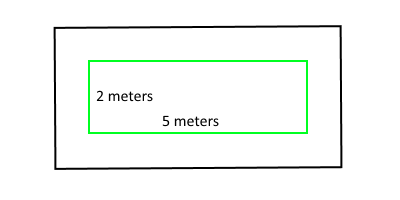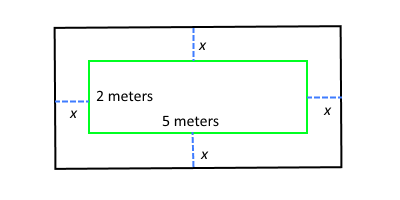### Home > CCA2 > Chapter 3 > Lesson 3.2.5 > Problem3-123

3-123.

Phana’s garden is $2$ meters wide and $5$ meters long. She puts a walkway of uniform width around her garden. If the area of the walkway is $30$ square meters, what are the outer dimensions of the walkway? Drawing a diagram will help you solve this problem.

Draw a diagram.

Rectangle, with green rectangle centered inside, left side labeled, 2 meters, bottom side labeled, 5 meters.

Find the total area of the outside rectangle by adding
the area of the inside rectangle to the area of the walkway.

$30+10=40\ \text{sq m}$

Added, blue dashed lines, perpendicular to sides, between top, bottom, right, & left sides, of inner & outer rectangles, each labeled, x.

If $x$ meters of walkway was added to each side, write an
expression for each side of the outside rectangle.

$\text{length}=5+2x$
$\text{width}=2+2x$Now write an equation of the form (length)(width) = area and solve for $x$.
Make sure you remember to answer the original question.